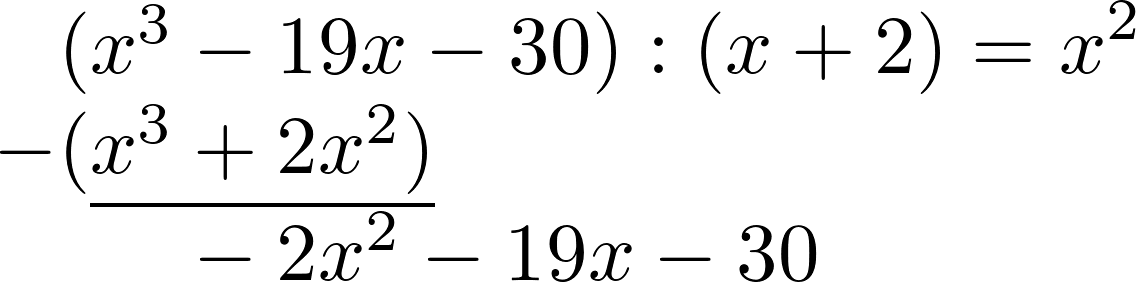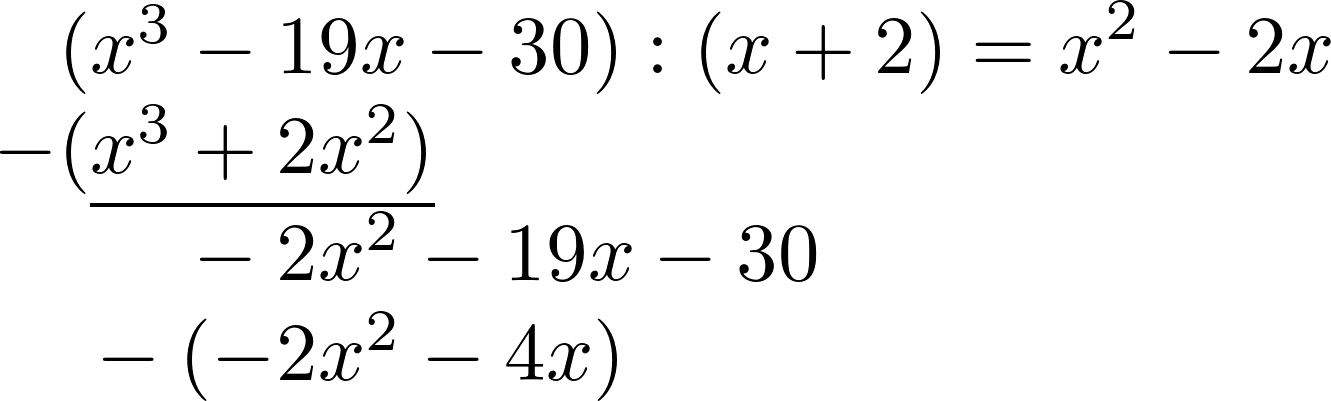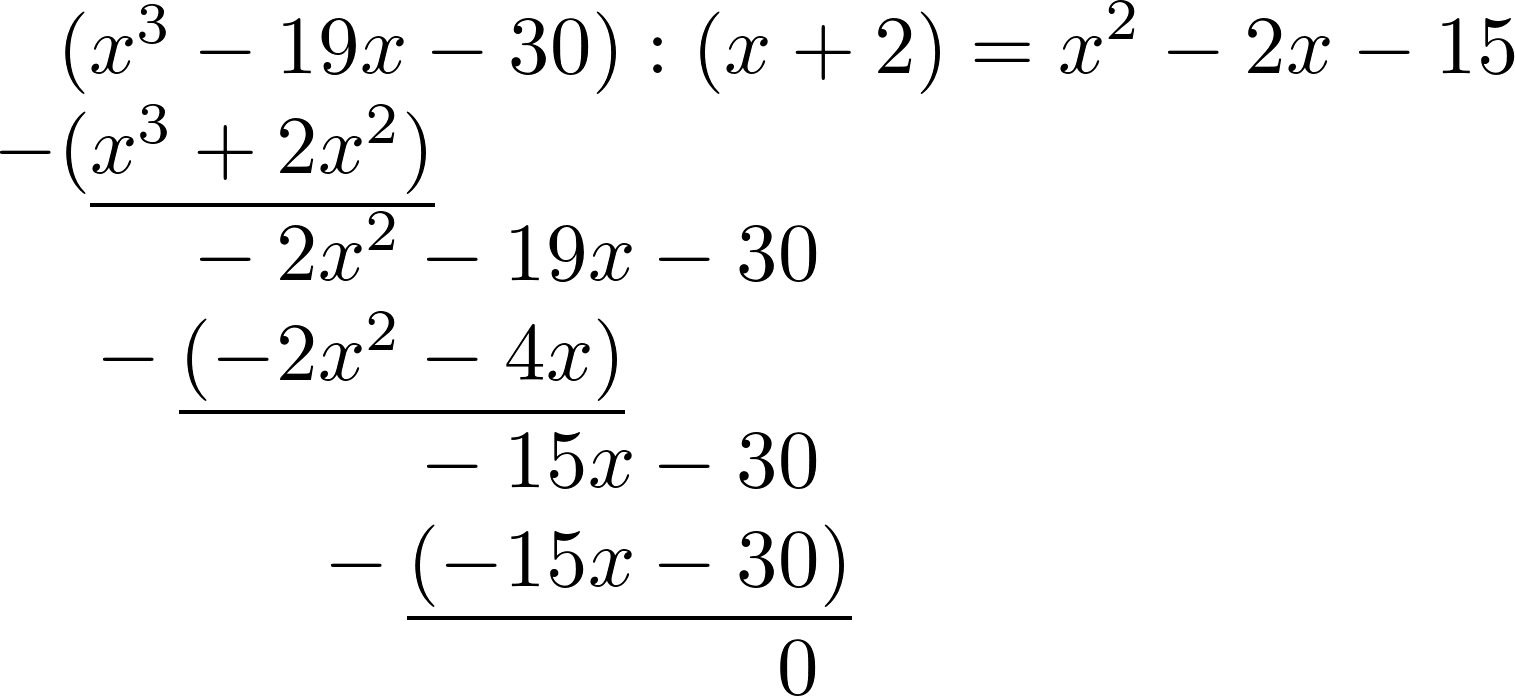Math Solve equations Polynomial long division

# Polynomial long division

Cubic equations are 3rd degree polynomial equations and have the form:

$ax^3+bx^2+cx+d=0$

In order to solve cubic equations and equations of higher degree one needs the Polynomial long division.

First, a zero is guessed by trial and error and then, using polynomial long division, one can transform the equation into a quadratic equation.

i

### Method

1. Guess a zero $x_N$
2. Polynomial long division: Divide equation by $(x-x_N)$
3. Solve the quadratic equation
i

### Hint

The quadratic equation obtained after polynomial division can be solved with the quadratic formula.

### Example

Solve cubic equation: $x^3-19x-30=0$

1. #### Guess a zero

The first zero must be found by trial and error.
Use different values for $x$ until 0 results.

$x^3-19x-30=0$

$x=1$:
$1^3-19\cdot1-30=-48$ $\neq0$ =>no zero

$x=-1$:
$(-1)^3-19\cdot(-1)-30=-12$ $\neq0$ =>no zero

$x=-2$:
$(-2)^3-19\cdot(-2)-30=-0$ =>zero at $x_{1}=-2$
2. #### Polynomial long division

The function is divided by $(x-x_1)$. Use polynomial long division for this.

$(x^3-19x-30):(x+2)$

First calculate $x^3:x$ and write down the result.Now $x^2$ is multiplied by $(x+2)$. The result is written in the second line and receives a minus.Both lines are now added together with the remainder written underneath.Similarly as before, one now calculates $-2x^2:x$. Write the result to the right and multiply it by $(x+2)$ to put it in the line below.The two lines are subtracted again.Finally, $-15x:x$ is calculated, multiplied back and deducted. The rest is 0 and the polynomial division is done.3. #### Solve quadratic equation

The new quadratic equation can now be solved e. g. with the pq-formula.
$x^2-2x-15=0$

$x_{2,3} = \frac{p}{2} \pm\sqrt{(\frac{p}{2})^2-q}$

$x_{2,3} = 1 \pm\sqrt{1^2+15}$
$x_{2,3} = 1 \pm\sqrt{16}$
$x_{2,3} = 1 \pm4$

$x_2=5$ and $x_3=-3$

The solutions of the initial equation are $x_1=-2$, $x_2=5$ and $x_3=-3$